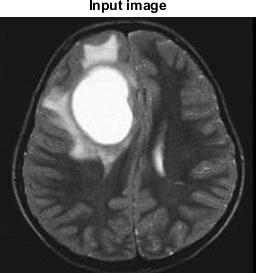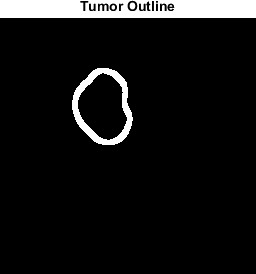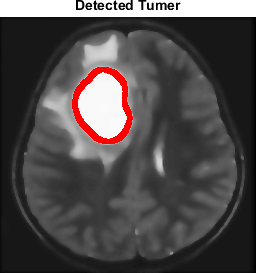• +91-9872993883
• +91-8283824812
• info@ris-ai.com

# Thesis Implementation Sample - Brain Tumor Segmentation in Matlab

% %%%
% % Brain Tumor Detection and Segmentation from MRI
%% For getting the function anisodiff, click on this link anisodiff
% % Brain Tumor is a fatal disease which cannot be confidently detected without MRI.
% In the project, it is tried to detect whether patient’s brain has tumor or not from MRI
% image using MATLAB simulation.
% To pave the way for morphological operation on MRI image, the image was first
% filtered using Anisotropic Diffusion Filter to reduce contrast between consecutive
% pixels. After that the image was resized and utilizing a threshold value image was
% converted to a black and white image manually. This primary filter the plausible
% locations for tumor presence.
% On this semi processed image morphological operations have been applied and
% information on solidity and areas of the plausible locations was obtained. A
% minimum value of both of this characters has been determined from statistical
% average of different MRI images containing tumor. Then it was used to deliver final
% detection result.
% Though this simulation routine can give correct result most of the time, it fails to
% perform when tumor’s size is too small or tumor is hollow.
% The larger goal of the project is to build a data base of 2D image data of tumor from
% the MRI images taken from different angle of a particular human and by analyzing
% them to point out the exact 3D location of the tumor . To fulfill this, 2D tumor
% detection and segmentation have been developed to better accuracy so that 3D
% detection can be more reliable. This is the primary target of the project. %%%
clc
close all
clear all

#### Input

% [I,path]=uigetfile('*.jpg','select a input image');
% str=strcat(path,I);
figure;
imshow(s);
title('Input image','FontSize',20);## Filter

num_iter = 10;
delta_t = 1/7;
kappa = 15;
option = 2;
disp('Preprocessing image please wait . . .');
Preprocessing image please wait . . .
inp = anisodiff(s,num_iter,delta_t,kappa,option);
Removing noise Filtering Completed !!
inp = uint8(inp);
inp=imresize(inp,[256,256]);
if size(inp,3)>1
inp=rgb2gray(inp);
end
figure;
imshow(inp);
title('Filtered image','FontSize',20);## thresholding

sout=imresize(inp,[256,256]);
t0=60;
th=t0+((max(inp(:))+min(inp(:)))./2);
for i=1:1:size(inp,1)
for j=1:1:size(inp,2)
if inp(i,j)>th
sout(i,j)=1;
else
sout(i,j)=0;
end
end
end

## Morphological Operation

label=bwlabel(sout);
stats=regionprops(logical(sout),'Solidity','Area','BoundingBox');
density=[stats.Solidity];
area=[stats.Area];
high_dense_area=density>0.6;
max_area=max(area(high_dense_area));
tumor_label=find(area==max_area);
tumor=ismember(label,tumor_label);
if max_area>100
figure;
imshow(tumor)
title('tumor alone','FontSize',20);
else
h = msgbox('No Tumor!!','status');
%disp('no tumor');
return;
end## Bounding box

box = stats(tumor_label);
wantedBox = box.BoundingBox;
figure
imshow(inp);
title('Bounding Box','FontSize',20);
hold on;
rectangle('Position',wantedBox,'EdgeColor','y');
hold off;## Getting Tumor Outline - image filling, eroding, subtracting

erosion the walls by a few pixels
dilationAmount = 5;
[r,c] = size(tumor);
filledImage = imfill(tumor, 'holes');
for i=1:r
for j=1:c
if x1<1
x1=1;
end
if x2>r
x2=r;
end
if y1<1
y1=1;
end
if y2>c
y2=c;
end
erodedImage(i,j) = min(min(filledImage(x1:x2,y1:y2)));
end
end
figure
imshow(erodedImage);
title('eroded image','FontSize',20);## subtracting eroded image from original BW image

tumorOutline=tumor;
tumorOutline(erodedImage)=0;
figure;
imshow(tumorOutline);
title('Tumor Outline','FontSize',20);## Inserting the outline in filtered image in green color

rgb = inp(:,:,[1 1 1]);
red = rgb(:,:,1);
red(tumorOutline)=255;
green = rgb(:,:,2);
green(tumorOutline)=0;
blue = rgb(:,:,3);
blue(tumorOutline)=0;
tumorOutlineInserted(:,:,1) = red;
tumorOutlineInserted(:,:,2) = green;
tumorOutlineInserted(:,:,3) = blue;
figure
imshow(tumorOutlineInserted);
title('Detected Tumer','FontSize',20);## Display Together

figure
subplot(231);imshow(s);title('Input image','FontSize',20);
subplot(232);imshow(inp);title('Filtered image','FontSize',20);
subplot(233);imshow(inp);title('Bounding Box','FontSize',20);
hold on;rectangle('Position',wantedBox,'EdgeColor','y');hold off;
subplot(234);imshow(tumor);title('tumor alone','FontSize',20);
subplot(235);imshow(tumorOutline);title('Tumor Outline','FontSize',20);
subplot(236);imshow(tumorOutlineInserted);title('Detected Tumor','FontSize',20);